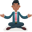Trusted answers to developer questions
Trusted Answers to Developer Questions

Related Tags

julia

# How to create a scatter plot in JuliaAbhilash

Grokking Modern System Design Interview for Engineers & Managers

Ace your System Design Interview and take your career to the next level. Learn to handle the design of applications like Netflix, Quora, Facebook, Uber, and many more in a 45-min interview. Learn the RESHADED framework for architecting web-scale applications by determining requirements, constraints, and assumptions before diving into a step-by-step design process.### Overview

In Julia, we can plot using various packages like pyplot, plotly and more. The Plots.jl is one such package that can be used to plot in Julia.

The Plots.jl can be installed using the following commands:

import Pkg;


The first command imports the package manager. The second command downloads and installs the package.

To use the package, execute the following command:

using Plots


### Scatter plot

In Julia, a scatter plot is created using the scatter() function of the Plots package.

### Syntax

using Plots

scatter(x, y)


### Parameters

• x: This is the data points along the x dimension.
• y: This is the data points along the y dimension.

### Code example

Let’s look at the code below:

using Plotsx = [1, 2, 3, 4, 5]y = [-1, 5, -3, 7, 2]scatter(x,y)savefig("output/plot.png")
The scatter plot

### Code explanation

• Line 1: We import the Plots package.
• Line 3: We define the x-dimension data points.
• Line 4: We define the y-dimension data points.
• Line 6: We create a scatter plot using the scatter() function.
• Line 7: We save the plot to the local disk as plot.png.

RELATED TAGS

julia

CONTRIBUTORAbhilash